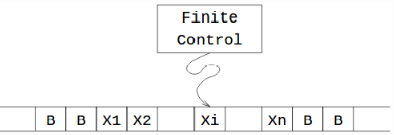Courses

# Test: Programming Techniques Storage And Subroutines

## 10 Questions MCQ Test Theory of Computation | Test: Programming Techniques Storage And Subroutines

Description
This mock test of Test: Programming Techniques Storage And Subroutines for Computer Science Engineering (CSE) helps you for every Computer Science Engineering (CSE) entrance exam. This contains 10 Multiple Choice Questions for Computer Science Engineering (CSE) Test: Programming Techniques Storage And Subroutines (mcq) to study with solutions a complete question bank. The solved questions answers in this Test: Programming Techniques Storage And Subroutines quiz give you a good mix of easy questions and tough questions. Computer Science Engineering (CSE) students definitely take this Test: Programming Techniques Storage And Subroutines exercise for a better result in the exam. You can find other Test: Programming Techniques Storage And Subroutines extra questions, long questions & short questions for Computer Science Engineering (CSE) on EduRev as well by searching above.
QUESTION: 1

### A turing machine has ____________ number of states in a CPU.

Solution:

A turing machine has finite number of states in its CPU. However the states are not small in number. Real computer consist of registers which can store values (fixed number of bits).

QUESTION: 2

### Suppose we have a simple computer with control unit holding a PC with a 32 bit address + Arithmetic unit holding one double length 64 bit Arithmetic Register. The number of states the finite machine will hold:

Solution:

According to the statistics of the question, we will have a finite machine with 2^96 states.

QUESTION: 3

### In one move a turing machine will:Solution:

A move of a turing machine is the function of the state of finite control and the tape symbol just scanned.

QUESTION: 4

Statement: We can use the finite control of turing machine to hold a finite amount of data.

Solution:QUESTION: 5

Statement 1: Multitrack Turing machine.
Statement 2: Gamma is Cartesian product of a finite number of finite sets.
Which among the following is the correct option?

Solution:

Cartesian product works like a struct in C/C++. For Example: Computer tape storage is something like 8 or 9 bits in each cell. One can recognize a multi track tape machine by looking at the transitions because each will have tuples as the read and write symbols.

QUESTION: 6

A multi track turing machine can described as a 6-tuple (Q, X, S, d, q0, F) where X represents:

Solution:

The 6-tuple (Q, X, S, d, q0, F) can be explained as:
Q represents finite set of states,
X represents the tape alphabet,
S represents the input alphabet
d represents the relation on states and the symbols
q0 represents the initial state
F represents the set of final states.

QUESTION: 7

Which of the following statements are false?

Solution:

In a n-track turing machine, one head reads and writes on all the tracks simultaneously.

QUESTION: 8

State true or false:Statement: Two track turing machine is equivalent to a standard turing machine.

Solution:

This can be generalized for n- tracks and can be proved equivalent using ennumerable languages.

QUESTION: 9

Which of the following is/are not true for recursively ennumerable language?

Solution:

In automata theory, a formal language is called recursively ennumerable language or partially decidable or semi decidable or turing acceptable or turing recognizable if there exists a turing machine which will ennumerate all valid strings of the language.

QUESTION: 10

According to Chomsky hierarchy, which of the following is adopted by Recursively Ennumerable language?

Solution:

Recursively Ennumerable languages are type 0 languages in the Chomsky hierarchy. All regular, context free, context sensitive languages are recursivelyennumerable language.